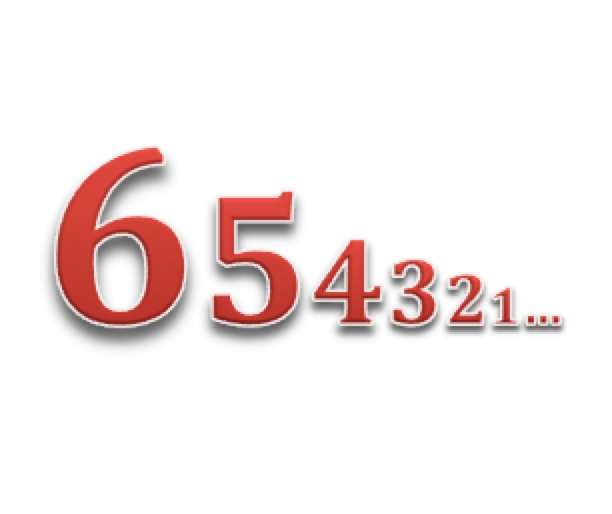# Prime Comic Numbers$\Large ...13121110987654321 \text{ is prime?}$

Let us define a comic number as one that is created by concatenating all of the integers from $1$ to $n$, left to right, in descending order. For example, if we let $n=12$, then our comic number would be $121110987654321$. What is the first value of $n$ such that its corresponding comic number is prime?

×